# Resources tagged with: Triangles

Filter by: Content type:
Age range:
Challenge level:

### There are 57 results

Broad Topics > Angles, Polygons, and Geometrical Proof > Triangles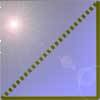### Cutting Corners

##### Age 7 to 11Challenge Level

Can you make the most extraordinary, the most amazing, the most unusual patterns/designs from these triangles which are made in a special way?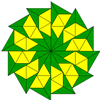### Triangle Shapes

##### Age 5 to 11Challenge Level

This practical problem challenges you to create shapes and patterns with two different types of triangle. You could even try overlapping them.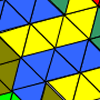### Tessellating Transformations

##### Age 7 to 11Challenge Level

Can you find out how the 6-triangle shape is transformed in these tessellations? Will the tessellations go on for ever? Why or why not?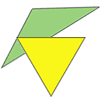### Triangle Relations

##### Age 7 to 11Challenge Level

What do these two triangles have in common? How are they related?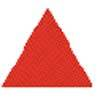### Tessellating Triangles

##### Age 7 to 11Challenge Level

Can you make these equilateral triangles fit together to cover the paper without any gaps between them? Can you tessellate isosceles triangles?### Tri.'s

##### Age 7 to 11Challenge Level

How many triangles can you make on the 3 by 3 pegboard?### Putting Two and Two Together

##### Age 7 to 11Challenge Level

In how many ways can you fit two of these yellow triangles together? Can you predict the number of ways two blue triangles can be fitted together?### More Transformations on a Pegboard

##### Age 7 to 11Challenge Level

Use the interactivity to find all the different right-angled triangles you can make by just moving one corner of the starting triangle.### Cut it Out

##### Age 7 to 11Challenge Level

Can you dissect an equilateral triangle into 6 smaller ones? What number of smaller equilateral triangles is it NOT possible to dissect a larger equilateral triangle into?### Uncanny Triangles

##### Age 7 to 11Challenge Level

Can you help the children find the two triangles which have the lengths of two sides numerically equal to their areas?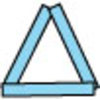### Sticks and Triangles

##### Age 7 to 11Challenge Level

Using different numbers of sticks, how many different triangles are you able to make? Can you make any rules about the numbers of sticks that make the most triangles?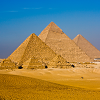### Egyptian Rope

##### Age 7 to 11Challenge Level

The ancient Egyptians were said to make right-angled triangles using a rope with twelve equal sections divided by knots. What other triangles could you make if you had a rope like this?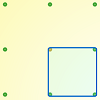### Peg and Pin Boards

##### Age 5 to 11

This article for teachers suggests activities based on pegboards, from pattern generation to finding all possible triangles, for example.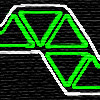### Tri-five

##### Age 7 to 11Challenge Level

Find all the different shapes that can be made by joining five equilateral triangles edge to edge.### Inside Seven Squares

##### Age 7 to 11Challenge Level

What is the total area of the four outside triangles which are outlined in red in this arrangement of squares inside each other?### Nine-pin Triangles

##### Age 7 to 11Challenge Level

How many different triangles can you make on a circular pegboard that has nine pegs?### Folding

##### Age 7 to 11Challenge Level

What shapes can you make by folding an A4 piece of paper?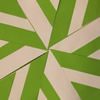### Folding Flowers 2

##### Age 7 to 11Challenge Level

Make a flower design using the same shape made out of different sizes of paper.### Triangles All Around

##### Age 7 to 11Challenge Level

Can you find all the different triangles on these peg boards, and find their angles?### Triangle Pin-down

##### Age 7 to 11Challenge Level

Use the interactivity to investigate what kinds of triangles can be drawn on peg boards with different numbers of pegs.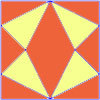### Fraction Fascination

##### Age 7 to 11Challenge Level

This problem challenges you to work out what fraction of the whole area of these pictures is taken up by various shapes.### Cut and Make

##### Age 7 to 11Challenge Level

Cut a square of paper into three pieces as shown. Now,can you use the 3 pieces to make a large triangle, a parallelogram and the square again?### Cutting it Out

##### Age 5 to 11Challenge Level

I cut this square into two different shapes. What can you say about the relationship between them?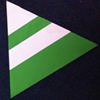### Regular Rings 1

##### Age 7 to 11Challenge Level

Can you work out what shape is made by folding in this way? Why not create some patterns using this shape but in different sizes?### Rectangle Tangle

##### Age 7 to 11Challenge Level

The large rectangle is divided into a series of smaller quadrilaterals and triangles. Can you untangle what fractional part is represented by each of the shapes?### Constructing Triangles

##### Age 11 to 14Challenge Level

Generate three random numbers to determine the side lengths of a triangle. What triangles can you draw?### Tetrahedra Tester

##### Age 11 to 14Challenge Level

An irregular tetrahedron is composed of four different triangles. Can such a tetrahedron be constructed where the side lengths are 4, 5, 6, 7, 8 and 9 units of length?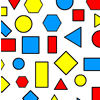### Sorting Logic Blocks

##### Age 5 to 11Challenge Level

This activity focuses on similarities and differences between shapes.### Bracelets

##### Age 7 to 11Challenge Level

Investigate the different shaped bracelets you could make from 18 different spherical beads. How do they compare if you use 24 beads?### Possible Pairs

##### Age 7 to 11Challenge Level

In this game, you turn over two cards and try to draw a triangle which has both properties.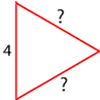### Number the Sides

##### Age 7 to 11Challenge Level

The triangles in these sets are similar - can you work out the lengths of the sides which have question marks?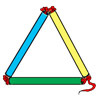### Making Maths: Test the Strength of a Triangle

##### Age 7 to 11Challenge Level

Have you noticed that triangles are used in manmade structures? Perhaps there is a good reason for this? 'Test a Triangle' and see how rigid triangles are.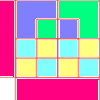### Diagrams

##### Age 7 to 11Challenge Level

A group activity using visualisation of squares and triangles.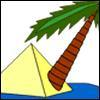### Triangle Island

##### Age 7 to 11Challenge Level

You have pitched your tent (the red triangle) on an island. Can you move it to the position shown by the purple triangle making sure you obey the rules?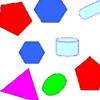### Part the Polygons

##### Age 7 to 11 ShortChallenge Level

Draw three straight lines to separate these shapes into four groups - each group must contain one of each shape.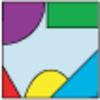### Jig Shapes

##### Age 5 to 11Challenge Level

Can you each work out what shape you have part of on your card? What will the rest of it look like?### Name That Triangle!

##### Age 7 to 11Challenge Level

Can you sketch triangles that fit in the cells in this grid? Which ones are impossible? How do you know?### Transformations on a Pegboard

##### Age 7 to 11Challenge Level

How would you move the bands on the pegboard to alter these shapes?### Isosceles Triangles

##### Age 11 to 14Challenge Level

Draw some isosceles triangles with an area of $9$cm$^2$ and a vertex at (20,20). If all the vertices must have whole number coordinates, how many is it possible to draw?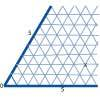### Cartesian Isometric

##### Age 7 to 11Challenge Level

The graph below is an oblique coordinate system based on 60 degree angles. It was drawn on isometric paper. What kinds of triangles do these points form?### Counting Triangles

##### Age 11 to 14Challenge Level

Triangles are formed by joining the vertices of a skeletal cube. How many different types of triangle are there? How many triangles altogether?### Property Chart

##### Age 11 to 14Challenge Level

A game in which players take it in turns to try to draw quadrilaterals (or triangles) with particular properties. Is it possible to fill the game grid?### Shapely Pairs

##### Age 11 to 14Challenge Level

A game in which players take it in turns to turn up two cards. If they can draw a triangle which satisfies both properties they win the pair of cards. And a few challenging questions to follow...### Making Maths: Equilateral Triangle Folding

##### Age 7 to 14Challenge Level

Make an equilateral triangle by folding paper and use it to make patterns of your own.### Triangle Transformation

##### Age 7 to 14Challenge Level

Start with a triangle. Can you cut it up to make a rectangle?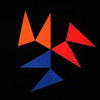### Notes on a Triangle

##### Age 11 to 14Challenge Level

Can you describe what happens in this film?### Dotty Triangles

##### Age 11 to 14Challenge Level

Imagine an infinitely large sheet of square dotty paper on which you can draw triangles of any size you wish (providing each vertex is on a dot). What areas is it/is it not possible to draw?### Triangular Tantaliser

##### Age 11 to 14Challenge Level

Draw all the possible distinct triangles on a 4 x 4 dotty grid. Convince me that you have all possible triangles.### Ratty

##### Age 11 to 14Challenge Level

If you know the sizes of the angles marked with coloured dots in this diagram which angles can you find by calculation?### Trice

##### Age 11 to 14Challenge Level

ABCDEFGH is a 3 by 3 by 3 cube. Point P is 1/3 along AB (that is AP : PB = 1 : 2), point Q is 1/3 along GH and point R is 1/3 along ED. What is the area of the triangle PQR?